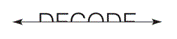Chapter 2.6, Problem 18E### Elementary Geometry for College St...

6th Edition
Daniel C. Alexander + 1 other
ISBN: 9781285195698

#### Solutions

Chapter
Section### Elementary Geometry for College St...

6th Edition
Daniel C. Alexander + 1 other
ISBN: 9781285195698
Textbook Problem
1 views

# Given that the horizontal line is a line of symmetry,complete each letter to discover the hidden word?To determine

To find:

The complete hidden word

Explanation

Given:

The given figure is,

Figure (1)

Definition Used:

If for every point A on the figure there is a second point A on the figure for which line l is the perpendicular bisector of AA¯, then the figure has symmetry with respect to horizontal line l.

Approach:

Consider various points on each letter of the word.

### Still sussing out bartleby?

Check out a sample textbook solution.

See a sample solution

#### The Solution to Your Study Problems

Bartleby provides explanations to thousands of textbook problems written by our experts, many with advanced degrees!

Get Started

#### What is the value of (X + 1) for the following scores: 0, 1, 4, 2? a. 8 b. 9 c. 11 d. 16

Essentials of Statistics for The Behavioral Sciences (MindTap Course List)

#### What is the partial fraction form of ?

Study Guide for Stewart's Single Variable Calculus: Early Transcendentals, 8th

#### Compute 8516453.

Mathematics For Machine Technology